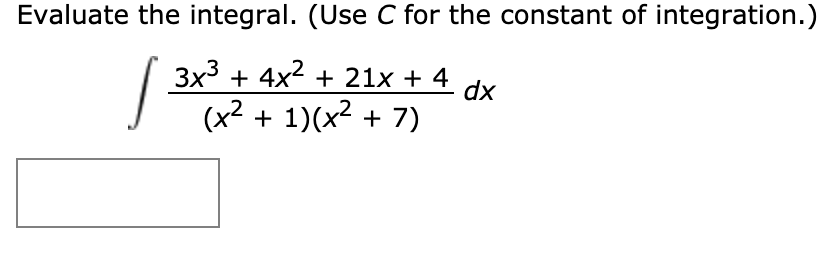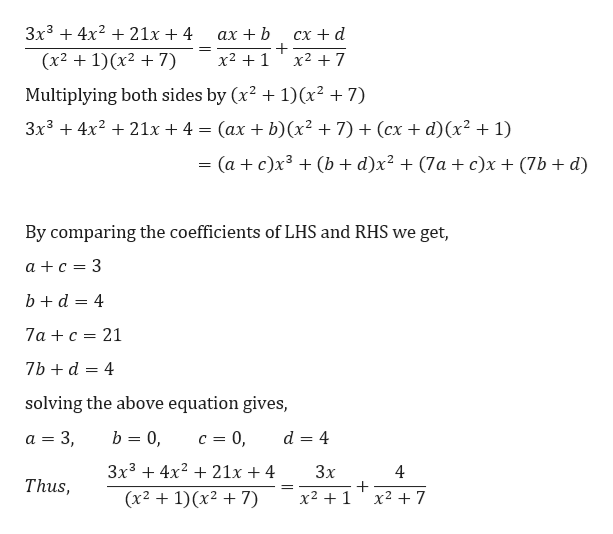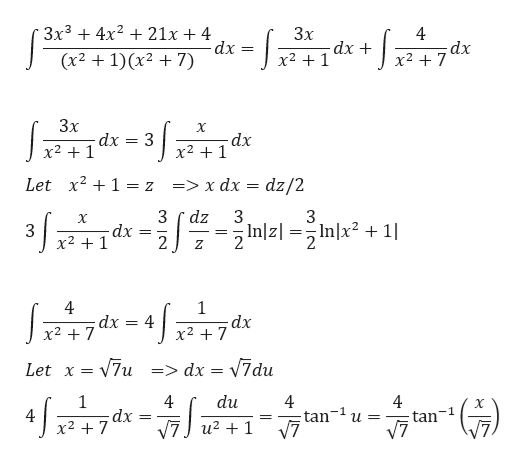Evaluate the integral. (Use C for the constant of integration.)3x3 4x221x + 4 dx(x21)(x27)

Question

How do you integrate

 3x3 + 4x2 + 21x + 4/ (x2 + 1)(x2 + 7)

by partial fractionshelp_outlineImage TranscriptioncloseEvaluate the integral. (Use C for the constant of integration.) 3x3 4x221x + 4 dx (x21)(x27) fullscreen
Step 1

We need to evaluate the given integral using partial fractions.

Step 2

We can proceed by finding the partial fractions ashelp_outlineImage Transcriptionclose3x34221x + 4 сх + d ах + b x21 (x2 1)x27) x2 7 1)(x2 +7) Multiplying both sides by (x2 3x3 21x + 4 (ах + b)(x? + 7) + (сх + d)(х? + 1) (ac)x3(b + d)x2 + (7a + c)x (7b d) and RHS we get, By comparing the coefficients of LH a c 3 bd 4 7ac 21 7bd 4 solving the above equation gives, b 0 а 3 3, c 0 d 4 3x34x 21x + 4 Зx 4 Thus, x21x2 7 (x21)(x27) fullscreen
Step 3

Hence,

...help_outlineImage Transcriptionclose-- 3x34x 21x 4 dx (x2 1)(x27) 3x dx x21 4 dx x27 3x dx x2 1 X dx x21 Let x2 1 = z => x dx = dz/2 dz Inlzl = 3 dx 2 S X 2Inzlnx? Inlx21 x2 1 Z 4 dx x2 7 4 x2 7 dx Let x = 7u=> dx = V7du 1 dx x2 7 4 du 4 tan u = V7 X -1 4 tan = _ fullscreen

Want to see the full answer?

See Solution

Want to see this answer and more?

Our solutions are written by experts, many with advanced degrees, and available 24/7

See Solution
Tagged in

Calculus